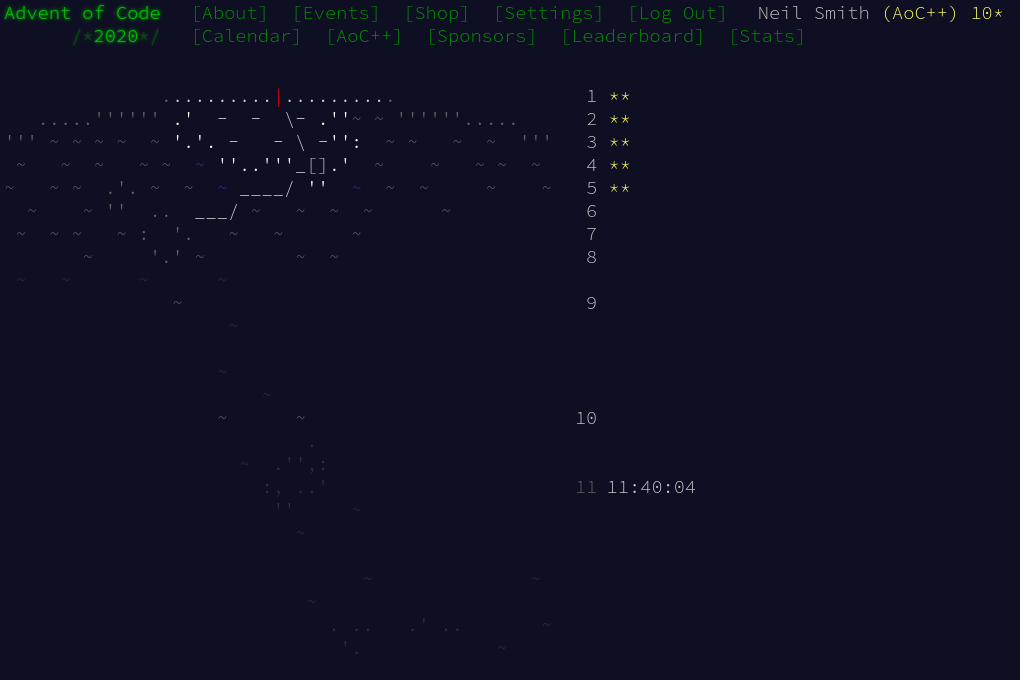December 12, 2020

# Advent of Code 2020 day 5

Starting with AttoparsecDay 5 was one of a complex description hiding a simple problem. All the guff about boarding numbers and the like obscured the fact that we were dealing with numbers written in base 2: binary. B and R represented the digit 1; F and L represented 0.

Haskell already has a function for converting strings of arbitrary "digits" in arbitrary bases into numbers: readInt. That needs a couple of supplementary functions for converting characters into digits, and it produces more output than we need, but the conversion is fairly painless.

directionToInt :: Char -> Int
directionToInt dir = if dir elem "BR" then 1 else 0

convert :: String -> Int
convert = fst . head . readInt 2 (elem "FBLR") directionToInt


Now I have essentially a list of numbers as input, finding the largest is trivial.

part1 = maximum . map convert


For part 2, finding the gap, I created the set of numbers I expected (in the range lowest to highest), and the set of numbers I had, subtracted one from the other, and that was the answer.

part2 passes = head \$ expecteds \\ ns
where ns = map convert passes
highest = maximum ns
lowest  = minimum ns
expecteds = [lowest..highest]


I could have used a Set, but the set-like operations in Data.List were sufficient for this case.

## Code

You can find the code here or on Gitlab.